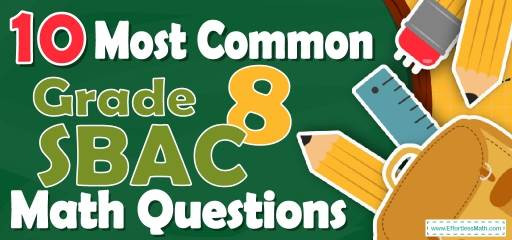# 10 Most Common 8th Grade SBAC Math QuestionsPreparing students for the 8th Grade SBAC Math test? Want a preview of the most common mathematics questions on the 8th Grade SBAC Math test? If so, then you are in the right place.

The mathematics section of 8th Grade SBAC can be a challenging area for many test-takers, but with enough patience, it can be easy and even enjoyable!

Preparing for the 8th Grade SBAC Math test can be a nerve-wracking experience for some students. Learning more about what they’re going to see when they take the 8th Grade SBAC can help to reduce those pre-test jitters. Here’s a chance to review the 10 most common 8th Grade SBAC Math questions to help your students know what to expect and what to practice most. Let your students try these 10 most common 8th Grade SBAC Math questions to hone their mathematical skills and to see if their math skills are up to date on what’s being asked on the exam or if they still need more practice.

Make sure to follow some of the related links at the bottom of this post to get a better idea of what kind of mathematics questions students need to practice.

## 10 Sample 8th Grade SBAC Math Practice Questions

1- A chemical solution contains $$4\%$$ alcohol. If there is 24 ml of alcohol, what is the volume of the solution?

A. 240 ml

B. 480 ml

C. 600 ml

D. 1200 ml

2- What is the area of the shaded region?

A. 31

B. 40

C. 64

D. 80

3- A $40 shirt now selling for$28 is discounted by what percent?

A. $$20 \%$$

B. $$30 \%$$

C. $$40 \%$$

D. $$60 \%$$

4- How much interest is earned on a principal of $5000 invested at an interest rate of $$5\%$$ for four years? A.$250

B. $500 C.$1000

D. $2000 5- A swimming pool holds 2,000 cubic feet of water. The swimming pool is 25 feet long and 10 feet wide. How deep is the swimming pool? Write your answer in the box below. ________ 6- The price of a car was$20,000 in 2014, $16,000 in 2015 and$12,800 in 2016. What is the rate of depreciation of the price of a car per year?

A. $$15 \%$$

B. $$20 \%$$

C. $$25 \%$$

D. $$30 \%$$

7- What is the area of the shaded region if the diameter of the bigger circle is 12 inches and the diameter of the smaller circle is 8 inches.

A. $$16 π$$

B. $$20 π$$

C. $$36 π$$

D. $$80 π$$

8- What is the area of an isosceles right triangle that has one leg that measures 6 cm? _________

9- A taxi driver earns $9 per 1-hour work. If he works 10 hours a day and in 1 hour he uses 2-liters petrol with price$1 for 1-liter. How much money does he earn in one day?

A. $90 B.$88

C. $70 D.$60

10- What is the solution of the following system of equations?
$$\frac{-x}{2}+ \frac{y}{4} = 1$$
$$\frac{-5y}{6}+ 2x = 4$$

A. $$x=48,y=22$$

B. $$x=50,y=20$$

C. $$x=20,y=50$$

D. $$x=22,y=48$$

## Best 8th Grade SBAC Math Prep Resource for 2022

1- C
$$4\%$$ of the volume of the solution is alcohol. Let $$x$$ be the volume of the solution.
Then: $$4\%$$ of $$x = 24 ml ⇒ 0.04 x = 24 ⇒ x = 24 ÷ 0.04 = 600$$

2- B
Use the area of rectangle formula $$(s = a × b)$$.
To find the area of the shaded region subtracts the smaller rectangle from the bigger rectangle.
$$S_{1} – S_{2} = (10 ft × 8ft) – (5ft × 8ft) ⇒ S_{1} – S_{2} = 40ft$$

3- B
Use the formula for Percent of Change
$$\frac{New \space Value-Old \space Value}{Old \space Value}× 100 \%$$
$$\frac{28-40}{40}× 100 \% = –30 \%$$(negative sign here means that the new price is less than old price).

4- C
Use simple interest formula:
I=prt
(I = interest, p = principal, r = rate, t = time)
$$I=(5000)(0.05)(4)=1000$$

5- 8
Use formula of rectangle prism volume.
$$V = (length) (width) (height) ⇒ 2000 = (25) (10) (height) ⇒ height = 2000 ÷ 250 = 8$$

6- B
Use this formula: Percent of Change
$$\frac{New \space Value-Old \space Value}{Old \space Value}× 100 %$$
$$\frac{16000-20000}{20000}× 100 %$$
$$= 20 \% \space and \space \frac{12800-16000}{16000}× 100 \% = 20 \%$$

7- B
To find the area of the shaded region subtract smaller circle from bigger circle.
$$S_{bigger}-S_{smaller} =π(r_{bigger})^2 -π(r_{smaller})^2⇒S_{bigger}-S_{smaller}=π(6)^2-π(4)^2$$
$$⇒ 36 π – 16π = 20 π$$

8- 18
$$a = 6 ⇒$$ area of the triangle is:
$$\frac{1}{2}(6×6)=\frac{36}{2}=18 \space cm^2$$

9- C
$$9×10=90$$
Petrol use: $$10×2=20 \space liters$$
Petrol cost: $$20×1=20$$
Money earned: $$90-20=70$$

10- D
$$\frac{-x}{2}+ \frac{y}{4} = 1$$
$$\frac{-5y}{6}+ 2x = 4$$
Multiply the top equation by 4. Then,
$$-2x+ y = 4$$
$$\frac{-5y}{6}+ 2x = 4$$
$$\frac{1}{6}y=8→y=48$$ , plug in the value of y into the first equation →$$x=22$$

## The Best Books to Ace 8th Grade SBAC Math Test

### What people say about "10 Most Common 8th Grade SBAC Math Questions - Effortless Math: We Help Students Learn to LOVE Mathematics"?

No one replied yet.

X
30% OFF

Limited time only!

Save Over 30%

SAVE $5 It was$16.99 now it is \$11.99